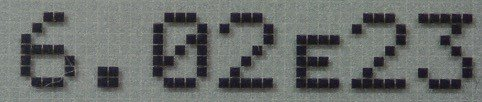Research Tweet

# What is Scientific Notation?

Scientific notation is a more appropriate way of writing small or very huge numbers.

The scientific notation helps us to signify the numbers which are very huge or very small in a form of multiplication of single-digit numbers and 10 raised to the power of the particular exponent.

The exponent is positive if the number given is very large and it is negative if the given number is very small.

## Scientific Notation Representation

The representation of scientific notation is given below;

a × 10b ; 1 ≤ a < 10

To express a number in scientific notation, move the decimal place to the right if the number given is less than zero or to the left if the given number is greater than zero.

For instance, in 456000, in this number the decimal present is after the last zero, therefore to express this in scientific notation, move the decimal to in between the numbers 4 and 5.

The decimal would move five places to the left to get 4.56 as per the representation of scientific notation mentioned above.

The number of times we move the decimal place develops the integer “b.” In the above-mentioned number, the decimal moved five times.

Therefore, the given number in scientific notation would be;4.56 × 105

### Scientific Notation Examples

Few Examples of scientific notation are given below;

1. 0.0007 = 7 x 10-4

2. 0.0345 = 3.45 x 10-2

3. 1234000 = 1.234 x 106

4. 8000 = 8 x 103

Another way used to write this expression, is to use the alphabet E which represents “times ten to the power of.”

For instance:Scientific notation for Avogadro’s number is also an example of scientific notation as shown above on a calculator. 6.02E23 which is the same as6.02 x 1023. This method is mostly used by computer programs and calculators.

Scientific notation enables us to compare between orders of magnitude given in the question.

For instance, 100000000 can be inscribed as 108 in scientific notation. the exponent here is positive. Likewise, 0.0000001 is a very small number and can be represented in scientific notation as 10-8, here the exponent written is negative.

#### Scientific Notation in Daily Life

Few examples of measurements where the scientific notation is useful are given below;

• The wavelength for violet light is 40-millionths centimeter which can be written in scientific notation as 4 × 10−5cm.

• Some black holes are measured by the number of solar masses they comprise. One black hole measured as 10,000,000 or in scientific notation as1.0 × 107 solar masses.

• A computer hard disk holds 4 gigabytes (about 4,000,000,000 bytes) of data. That is written in scientific notation as 4.0 × 109 bytes.

• Computer calculation speeds are frequently measured in nanoseconds. A nanosecond is equal to 0.000000001 seconds or can be written in scientific notation as 1.0 × 10−9 seconds.

##### Rules for Scientific Notation

To determine the power or exponent of 10, the following rules must be followed:

• The base must be 10

• The exponent should be a non-zero integer, which means it can be either be written in positive or negative integer

• The absolute value of the coefficient is either bigger than or equal to 1 nonetheless it should be less than 10.

• Coefficients can either be positive or negative figures including whole and decimal numbers.

###### Positive and Negative Exponent of Scientific Notation

When the scientific notation of any huge numbers is stated, then use the positive exponents for base 10.

For instance: 80000 = 8 x 104, where 4 is expressed as the positive exponent.

When the scientific notation of any small numbers is stated, then the use of negative exponents is used for base 10.

For instance: 0.0006 = 6 x 10-4, where -4 is expressed as the negative exponent.

In simple terms, it can be said that the number larger than 1 can be written as the expression with a positive exponent, while the numbers which are less than 1 can be expressed with a negative exponent.

###### Scientific Notation Question

Question 1: Convert 0.00000089 into scientific notation.

Solution: We have to move the decimal point to the right of the number 0.00000089 up to 7 places.

The decimal point thus moved 7 places to the right to form figure 8.9

Since the numbers given are less than 10 and the decimal is thus moved to its right.

Therefore, a negative exponent is used here.

⇒ 0.00000089 = 8.9 × 10-7

The above mentioned number is thus written in scientific notation.

Share

###### Similar Post: